# Area Of A Segment

Here we will learn about the area of a segment including how to identify a segment of a circle and how to find the area of it.

There are also worksheets based on Edexcel, AQA and OCR exam questions, along with further guidance on where to go next if you’re still stuck.

## What is the area of a segment?

The area of a segment is the space inside the segment that is formed by an arc and a chord.

A segment of a circle is the area enclosed by a chord and an arc of a circle. There are two types of segments,

‐ A minor segment is a segment where the arc length is less than half of the circumference of the circle.

‐ A major segment is a segment where the arc length is greater than half of the circumference of the circle.

To work out the area of a segment we can use the formula for the area of a triangle and the formula for the area of a sector. We subtract the area of the triangle from the area of the sector.

The formula for the area of a triangle is

\text { Area of a triangle }=\cfrac{\text { base } \times \text { height }}{2}.

Step-by-step guide: Area of a triangle

Higher – There is also a trigonometric formula we can use to find the area of a triangle, two sides and the included angle. Useful for triangles where we don’t know the height and we are unable to calculate it.

\text { Area of a triangle }=\cfrac{1}{2} \, a b \sin C

Step-by-step guide: Area of a triangle trig = (\cfrac{1}{2} \, a b \sin C)

The formula for the area of a sector is

\text { Area of a sector }=\cfrac{\theta}{360} \, \times \pi r^2

where \theta is the angle of the sector and r is the radius of the circle.

Step-by-step guide: Area of a sector

### What is the area of a segment?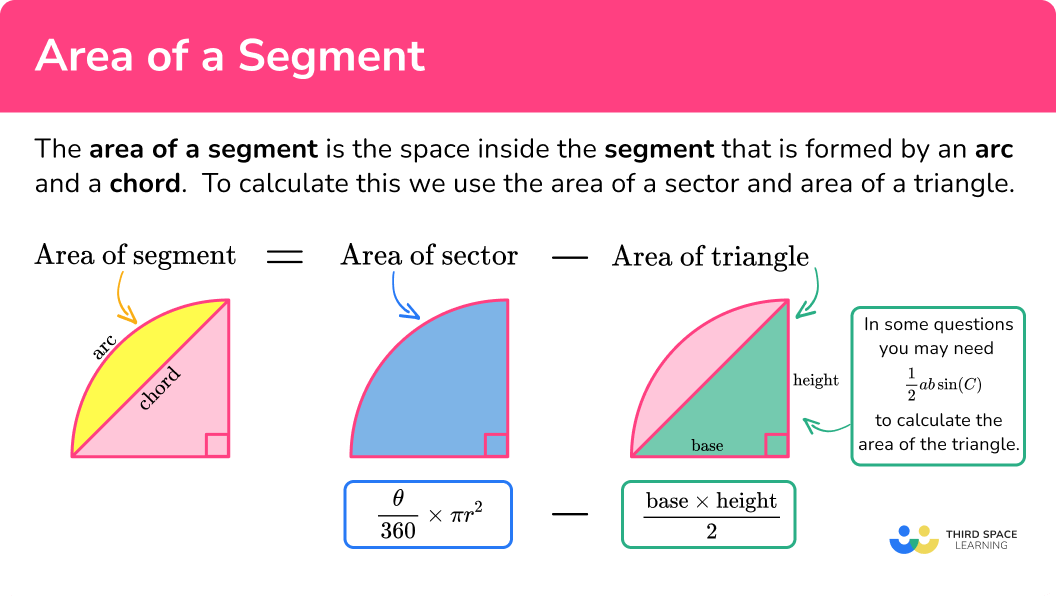## How to calculate the area of a segment

In order to calculate the area of a segment:

1. Find the length of the radius.
2. Find the size of the angle creating the sector.
3. Find the area of the sector.
4. Find the area of the triangle created by the radii and the chord of a circle.
5. Subtract the area of the triangle from the area of the sector.

### Explain how to calculate the area of a segment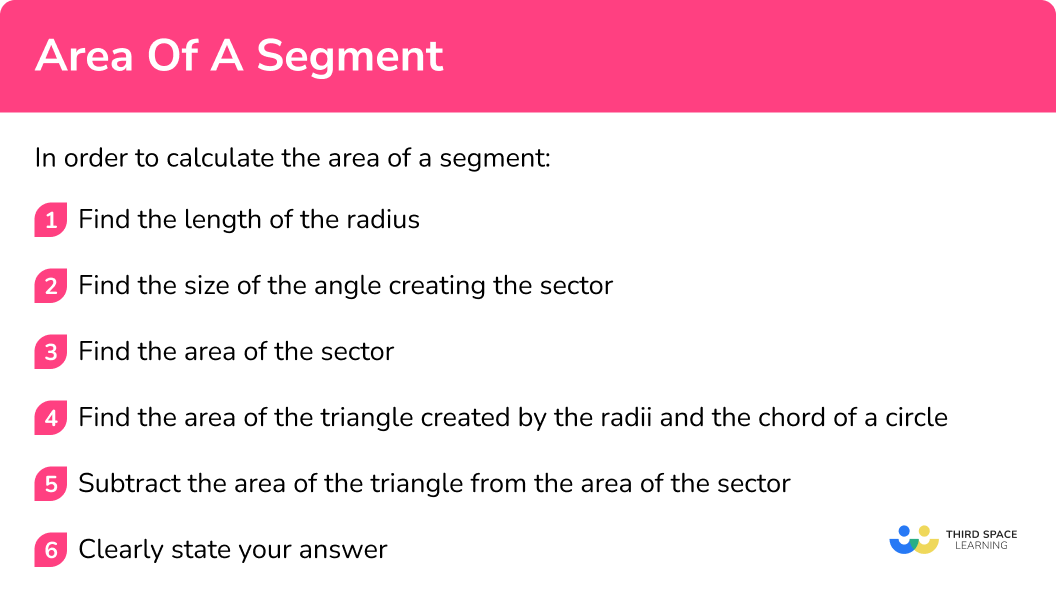Area of a segment is part of our series of lessons to support revision on circles, sectors and arcs. You may find it helpful to start with the main circles, sectors and arcs lesson for a summary of what to expect, or use the step by step guides below for further detail on individual topics. Other lessons in this series include:

## Area of a segment examples

### Example 1: using area of a triangle formula

1. Find the length of the radius.

The length of the radius is labelled on the diagram.

2Find the size of the angle creating the sector.

The angle creating the sector is marked on the diagram.

\theta=90^{\circ}

3Find the area of the sector.

The whole shape is the sector.

The area of the sector is

\begin{aligned} & =\cfrac{\theta}{360} \, \times \pi r^2 \\\\ & =\cfrac{90}{360} \, \times \pi \times 3^2 \\\\ & =\cfrac{9}{4} \, \pi \\\\ & =7.0685… \mathrm{~cm}^2 \end{aligned}

Note: We could have found the area of the sector, in this instance, by using the area of a circle formula to find the area of the circle as a whole, and then divided by four, because this sector has a 90^{\circ} angle, which means it is one quarter of the whole circle.

4Find the area of the triangle created by the radii and the chord of a circle.

The triangle created by the radii and the chord of the circle is the white sections of the sector. It is a right-angled triangle.

The area of the triangle is

\begin{aligned}& =\cfrac{\text{ base } \times \text{ height }}{2} \\\\ & =\cfrac{3 \, \times \, 3}{2} \\\\ & =4.5 \mathrm{~cm}^2\end{aligned}

5Subtract the area of the triangle from the area of the sector.

The area of the shaded area, which is the segment, is

=7.0685…-4.5=2.5685…

=2.57 \mathrm{~cm}^2 .

### Example 2: using area of a triangle formula

The length of the radius is labelled on the diagram.

The angle creating the sector is marked on the diagram.

\theta=90^{\circ}

The whole shape is the sector.

The area of the sector is

\begin{aligned}& =\cfrac{\theta}{360} \times \pi r^2 \\\\ & =\cfrac{90}{360} \times \pi \times 5^2 \\\\ & =\cfrac{25}{4} \pi \\\\ & =19.63495408… \mathrm{~cm}^2\end{aligned}

The triangle created by the radii and the chord of the circle is the white sections of the sector.

The area of the triangle is

\begin{aligned}& =\cfrac{\text { base } \times \text { height }}{2} \\\\ & =\cfrac{5 \times 5}{2} \\\\ & =12.5 \mathrm{~cm}^2\end{aligned}

The area of the shaded area, which is the segment, is

=19.63495408…-12.5

=7.134954085…

The question asks for the answer rounded to 2 decimal places, so we can round it and give the answer as 7.13 \mathrm{~cm}^2.

### Example 3: using trigonometry

The length of the radius is labelled on the diagram.

The angle creating the sector is marked on the diagram.

\theta=108^{\circ}

The area of the sector is

\begin{aligned}& =\cfrac{\theta}{360} \times \pi r^2 \\\\ & =\cfrac{108}{360} \times \pi \times 4^2 \\\\ & =\cfrac{24}{5} \pi \\\\ & =15.07964474… \mathrm{~cm}^2\end{aligned}

We do not know the height of the triangle created by the radii and the chord of the circle in this example.

So, we use trigonometry for the area of a triangle, \text { Area of a triangle }=\cfrac{1}{2} \, a b \sin C, where a and b are radii, and the angle C is the angle creating the sector.

The area of the triangle is

\begin{aligned}& =\cfrac{1}{2} \, a b \sin C \\\\ & =\cfrac{1}{2} \times 4 \times 4 \times \sin (108) \\\\ & =7.60845213… \mathrm{~cm}^2\end{aligned}

The area of the shaded area, which is the segment, is

=15.07964474… - 7.60845213…

= 7.47119261…

The question asks for the answer rounded to 3 significant figures, so we can round it and give the answer as 7.47 \mathrm{~cm}^2.

### Example 4: theta not given

Below is a circle with radius 6 \, mm. Calculate the area of the shaded segment. Give your answer to 3 significant figures.

The length of the radius is given in the question.

The angle creating the sector is not marked on the diagram. However, we can use the angle fact ‘angles around a point add to 360^{\circ} ’ to calculate the angle creating the sector.

\theta=360-246=114^{\circ}

The area of the sector is

\begin{aligned}& =\cfrac{\theta}{360} \times \pi r^2 \\\\ & =\cfrac{114}{360} \times \pi \times 6^2 \\\\ & =\cfrac{57}{5} \pi \\\\ & =35.8145625… \mathrm{~mm}^2\end{aligned}

We do not know the height of the triangle created by the radii and the chord of the circle in this example.

So, we use the trigonometric formula for the area of a triangle, \text { Area of a triangle }=\cfrac{1}{2} \, a b \sin C, where a and b are radii, and the angle C is the angle creating the sector.

The area of the triangle is

\begin{aligned}& =\cfrac{1}{2} \, a b \sin C \\\\ & =\cfrac{1}{2} \times 6 \times 6 \times \sin (114) \\\\ & =16.44381824 \mathrm{~mm}^2\end{aligned}

The area of the shaded area, which is the segment, is

=35.8145625… - 16.44381824…

=19.37074426…

The question asks for the answer rounded to 3 significant figures, so we can round it and give the answer as 19.4 \mathrm{~mm}^2.

### Example 5: given base angle of isosceles triangle

Below is a circle with radius 4 \, mm. Calculate the area of the shaded segment. Give your answer to 3 significant figures.

The length of the radius is given in the question.

The angle creating the sector is not marked on the diagram. We need to calculate it. The first thing to recognise is which sides of the triangle formed in the sector are radii and which is the chord.

The two sides that meet in the centre of the circle are both radii, therefore, equal in length. The third side of the triangle cuts the circle from circumference to circumference, not passing through the centre, so is the chord.

The fact that we have two equal sides in this triangle, means it is isosceles.

The angle facts ‘base angles in an isosceles triangle are equal’ and ‘angles in a triangle add to 180^{\circ} ’ will help us to calculate the angle creating the sector, \theta .

\theta=180-22-22=136^{\circ}

The area of the sector is

\begin{aligned}& =\cfrac{\theta}{360} \times \pi r^2 \\\\ & =\cfrac{136}{360} \times \pi \times 4^2 \\\\ & =\cfrac{272}{45} \pi \\\\ & =18.98918226… \mathrm{~mm}^2\end{aligned}

We do not know the height of the triangle created by the radii and the chord of the circle in this example.

So, we use the area formula \text{ Area of a triangle }=\cfrac{1}{2} \, a b \sin C, where a and b are radii, and the angle C is the angle creating the sector.

The area of the triangle is

\begin{aligned}& =\cfrac{1}{2} \, ab \sin C \\\\ & =\cfrac{1}{2} \times 4 \times 4 \times \sin (136) \\\\ & =5.557266964… \mathrm{~mm}^2\end{aligned}

The area of the shaded area, which is the segment, is

=18.98918226… - 5.557266964…

=13.4319153…

The question asks for the answer rounded to 3 significant figures, so we can round it and give the answer as 13.4 \mathrm{~mm}^2.

Below is a sector. The area of the sector is 67.02 {~cm}^2. The radius of the sector, r, is an integer.

Calculate the area of the segment labelled B. Give your answer to 3 significant figures.

The length of the radius is given in the question as r. So we need to calculate it.

We are given the area of the sector, so we can use this to calculate the radius.

Substituting what we know into the formula for the area of a sector we get

67.02=\cfrac{120}{360} \times \pi \times r^2.

Rearranging this to make r^2 the subject gives

r^2=\cfrac{67.02}{\cfrac{120}{360} \times \pi} \, .

So,

r^2=63.99938572…

We want the radius, r, so we need to square root r^2, to give

r=7.999961607…

The question tells us that the radius, r, is an integer, so for this example we can say, r=8 \mathrm{~cm}.

The angle creating the sector is marked on the diagram.

\theta=120^{\circ}

The area of the sector is given in the question as 67.02 \mathrm{~cm}^2.

We do not know the height of the triangle created by the radii and the chord of the circle in this example.

So, we use the area formula \text{ Area of a triangle }=\cfrac{1}{2} \, a b \sin C, where a and b are radii, and the angle C is the angle creating the sector.

The area of the triangle is

\begin{aligned}& =\cfrac{1}{2} \, a b \sin C \\\\ & =\cfrac{1}{2} \times 8 \times 8 \times \sin (120) \\\\ & =27.71281292… \mathrm{~cm}^2\end{aligned}

The area of the shaded area, which is the segment, is

=67.02 - 27.71281292…

=39.30718708…

The question asks for the answer rounded to 3 significant figures, so we can round it and give the answer as 39.3 \mathrm{~cm}^2.

### Common misconceptions

• Sector and segment
Sometimes the two can be confused. Remember,
A sector is formed when a circle is divided using two radii.

A segment is the area enclosed by an arc of a circle and a chord.

• Recognising the angle creating the sector
The angle creating the sector is the angle inside the sector you’re working with.
For example, in the diagram below, it is the angle labelled 112^{\circ} that creates the sector, not the angle labelled 248^{\circ}.

• Rounding too early

### Practice area of a segment questions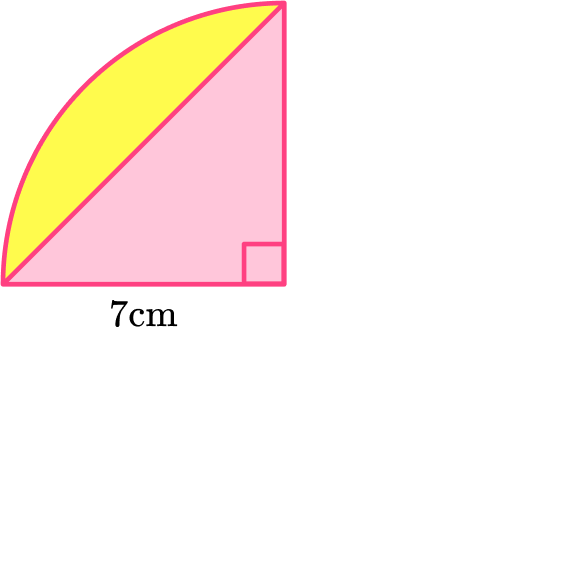14 \mathrm{~cm}^213.98 \mathrm{~cm}^262.98 \mathrm{~cm}^263 \mathrm{~cm}^2We need to calculate the area of the sector and the area of the triangle. Then subtract the area of the triangle from the area of the sector.

The area of the sector is

\begin{aligned}& =\cfrac{\theta}{360} \times \pi r^2 \\\\ & =\cfrac{90}{360} \times \pi \times 7^2 \\\\ & =\cfrac{49}{4} \pi \\\\ & =38.48451001… \mathrm{~cm}^2\end{aligned}

The area of the triangle is

\begin{aligned}& =\cfrac{\text { base } \times \text { height }}{2} \\\\ & =\cfrac{7 \times 7}{2} \\\\ & =24.5 \mathrm{~cm}^2\end{aligned}

Hence, the area of the segment is

=38.48451001…-24.5=13.98451001…=13.98 \; (2 d p).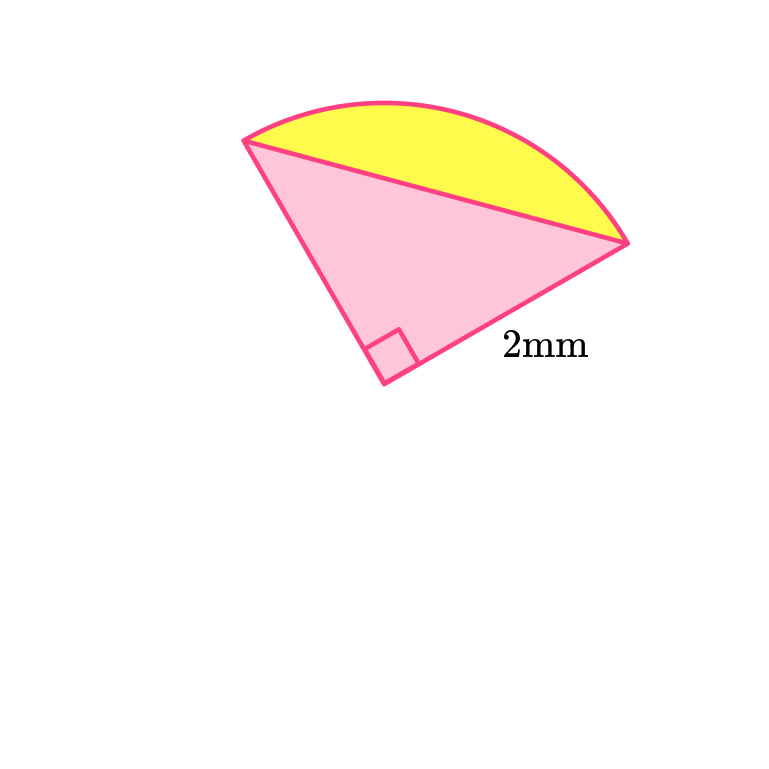5.14 \mathrm{~mm}^25 \mathrm{~mm}^21 \mathrm{~mm}^21.14 \mathrm{~mm}^2We need to calculate the area of the sector and the area of the triangle. Then subtract the area of the triangle from the area of the sector.

The area of the sector is

\begin{aligned}& =\cfrac{\theta}{360} \times \pi r^2 \\\\ & =\cfrac{90}{360} \times \pi \times 2^2 \\\\ & =\pi \\\\ & =3.141592654… \mathrm{~mm}^2\end{aligned}

The area of the triangle is

\begin{aligned} & =\cfrac{\text { base } \times \text { height }}{2} \\\\ & =\cfrac{2 \times 2}{2} \\\\ & =2 \mathrm{~mm}^2\end{aligned}

Hence, the area of the segment is

=3.141592654…-2=1.141592654…=1.14 \; (2 d p).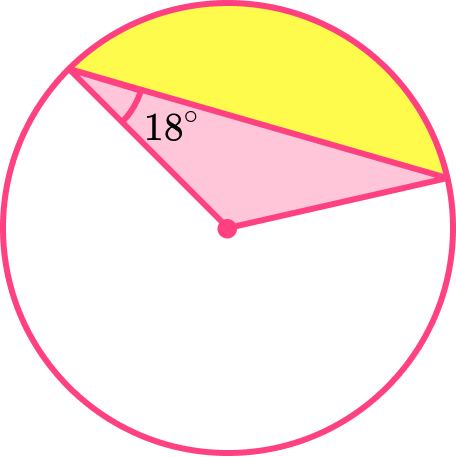77.98 \mathrm{~mm}^2179.77 \mathrm{~mm}^2178 \mathrm{~mm}^278 \mathrm{~mm}^2We need to calculate the area of the sector and the area of the triangle. Then subtract the area of the triangle from the area of the sector.

First we need to calculate \theta, the angle that creates the sector.

The two sides that meet in the centre of the circle are both radii, therefore, equal in length. The third side of the triangle cuts the circle from circumference to circumference, not passing through the centre, so is the chord.

The fact that we have two equal sides in this triangle, means it is isosceles.

The angle facts ‘base angles in an isosceles triangle are equal’ and ‘angles in a triangle add to 180^{\circ} ’ will help us to calculate the angle creating the sector, \theta.

\theta=180-18-18=144^{\circ}

The area of the sector is

\begin{aligned}& =\cfrac{\theta}{360} \times \pi r^2 \\\\ & =\cfrac{144}{360} \times \pi \times 9^2 \\\\ & =\cfrac{162}{5} \pi \\\\ & =101.787602 \mathrm{~mm}^2\end{aligned}

The area of the triangle is

\begin{aligned}& =\cfrac{1}{2} \, a b \sin C \\\\ & =\cfrac{1}{2} \times 9 \times 9 \times \sin (144) \\\\ & =23.80530272… \mathrm{~mm}^2\end{aligned}

Hence, the area of the segment is

=101.787602…-23.80530272…=77.98229928…=77.98 \; (2 d p).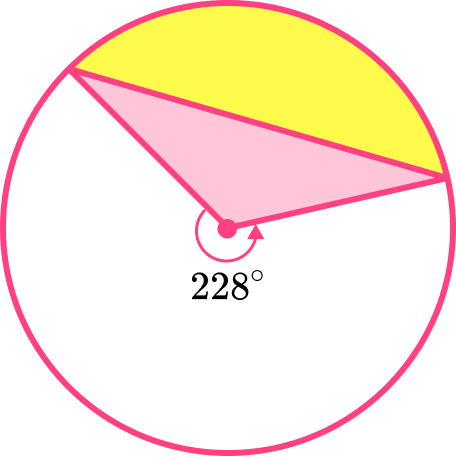17 \mathrm{~cm}^27.02 \mathrm{~cm}^217.39 \mathrm{~cm}^27 \mathrm{~cm}^2We need to calculate the area of the sector and the area of the triangle. Then subtract the area of the triangle from the area of the sector.

First we need to calculate \theta, the angle that creates the sector.

We can use the angle fact ‘angles around a point add to 360^{\circ} ’ to calculate the angle creating the sector.

\theta=360-228=132^{\circ}

The area of the sector is

\begin{aligned}& =\cfrac{\theta}{360} \times \pi r^2 \\\\ & =\cfrac{132}{360} \times \pi \times 3^2 \\\\ & =\cfrac{33}{10} \pi \\\\ & =10.36725576 \mathrm{~cm}^2\end{aligned}

The area of the triangle is

\begin{aligned}& =\cfrac{1}{2} \, a b \sin C \\\\ & =\cfrac{1}{2} \times 3 \times 3 \times \sin (132) \\\\ & =3.344151715… \mathrm{~cm}^2\end{aligned}

Hence, the area of the segment is

=10.36725576…-3.344151715…=7.023104045…=7.02 \; (2 d p).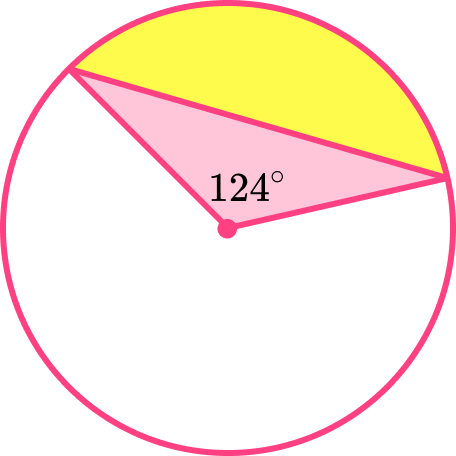43.74 \mathrm{~cm}^244 \mathrm{~cm}^217 \mathrm{~cm}^216.69 \mathrm{~cm}^2We need to calculate the area of the sector and the area of the triangle. Then subtract the area of the triangle from the area of the sector.

The area of the sector is

\begin{aligned}& =\cfrac{\theta}{360} \times \pi r^2 \\\\ & =\cfrac{124}{360} \times \pi \times 5^2 \\\\ & =\cfrac{155}{18} \pi \\\\ & =27.05260341 \mathrm{~cm}^2\end{aligned}

The area of the triangle is

\begin{aligned}& =\cfrac{1}{2} \, a b \sin C \\\\ &=\cfrac{1}{2} \times 5 \times 5 \times \sin (124) \\\\ & =10.36296966… \mathrm{~cm}^2\end{aligned}

Hence, the area of the segment is

=27.05260341…-10.36296966…=16.68963375…=16.69 \; (2 d p).

6. Below is a sector. The area of the sector is 33.93 {~cm}^2. The radius of the sector, r, is an integer. Calculate the area of the segment labelled B. Give your answer to 3 significant figures.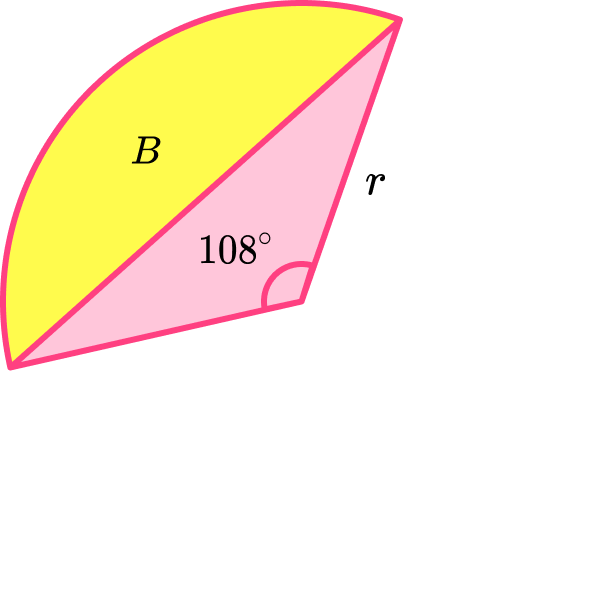16.81 \mathrm{~cm}^251.05 \mathrm{~cm}^251 \mathrm{~cm}^217 \mathrm{~cm}^2We need to calculate the area of the sector and the area of the triangle. Then subtract the area of the triangle from the area of the sector.

The length of the radius is given in the question as r. So we need to calculate it. We are given the area of the sector, so we can use this to calculate the radius.

Substituting what we know into the formula for the area of a sector we get

33.93=\cfrac{108}{360} \times \pi \times r^2 .

Rearranging this to make r^2 the subject gives

r^2=\cfrac{33.93}{\frac{108}{360} \times \pi} .

So,

r^2=36.00084813…

We want the radius, r, so we need to square root r^2, to give

r=6.000070677…

The question tells us that the radius,   r, is an integer, so we can say,   r=6 \mathrm{~cm}.

The area of the sector is given in the question. So we need to find the area of the triangle.

The area of the triangle is

\begin{aligned}& =\cfrac{1}{2} \, a b \sin C \\\\ & =\cfrac{1}{2} \times 6 \times 6 \times \sin (108) \\\\ & =17.11901729 \mathrm{~cm}^2\end{aligned}

Hence, the area of the segment is

=33.93-17.11901729…=16.81098271…=16.81 \; (2 d p).

### Area of a segment GCSE questions

1. The diagram shows a sector of a circle with centre Ο.

The radius of the circle is 8 \, cm.

ABC is an arc of the circle.

AC is a chord of the circle.

Angle AOC = 50^{\circ}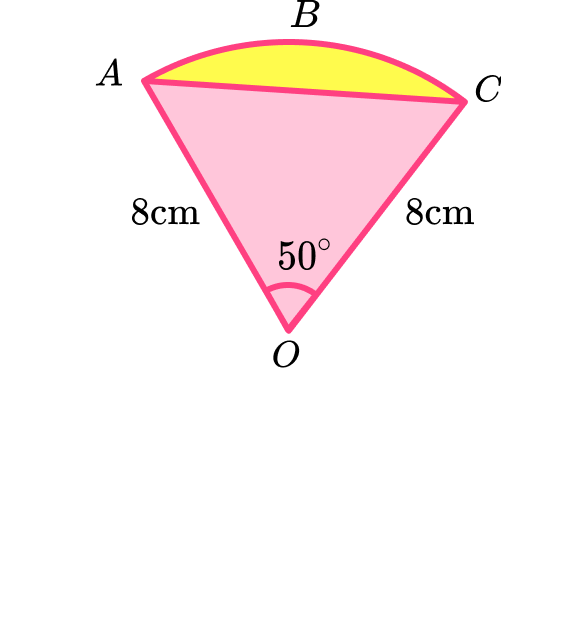Calculate the area of the shaded segment.

(5 marks)

\cfrac{50}{360}\times \pi \times 8^2 \, or \, \cfrac{1}{2}\times 8\times 8\times \sin(50)

(1)

Area of sector = 27.92

(1)

Area of triangle = 24.51…

(1)

‘Their area of sector’ – ’their area of triangle’.

(1)

3.41(…) {~cm}^2

(1)

2.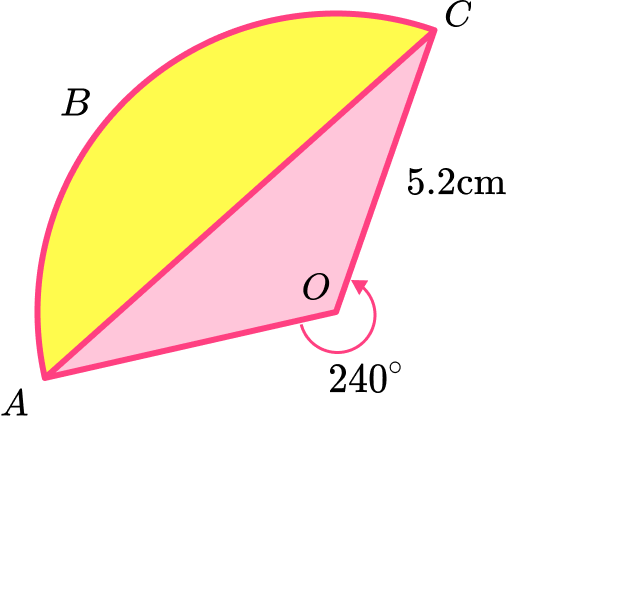The diagram shows sector Ο ABC of a circle with centre Ο.

OC = 5.2 \, cm

Calculate the area of the shaded segment ABC.

(6 marks)

360-240

(1)

120^{\circ}

(1)

\cfrac{120}{360}\times \pi \times 5.2^2 \, or \, \cfrac{1}{2}\times 5.2\times 5.2\times \sin(120)

(1)

Area of sector = 28.31… and area of triangle = 11.7

(1)

‘Their area of sector’ – ’their area of triangle’.

(1)

16.6(…) {~cm}^2

(1)

3. ABC is an arc of a circle centre O with radius 60 \, m.

AC is a chord of the circle.

Angle AOC = 35^{\circ}.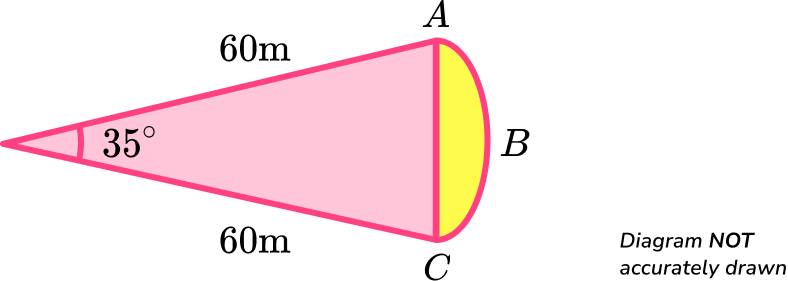Calculate the area of the shaded region.

(5 marks)

\cfrac{35}{360}\times \pi \times 60^2 \, or \, \cfrac{1}{2}\times 60\times 60\times \sin(35)

(1)

Area of sector = 1099.5(…)

(1)

Area of triangle = 1032.43(…)

(1)

‘Their area of sector’ – ’their area of triangle’.

(1)

67.1 {~m}^2

(1)

## Learning checklist

You have now learned how to:

• Calculate the area of a segment given the angle creating the sector, \theta , and the radius, r
• Calculate the radius given the area of the sector
• Recognise isosceles triangles in sectors
• Use the properties of isosceles triangles to find the angle creating the sector, \theta

## Still stuck?

Prepare your KS4 students for maths GCSEs success with Third Space Learning. Weekly online one to one GCSE maths revision lessons delivered by expert maths tutors.

Find out more about our GCSE maths tuition programme.